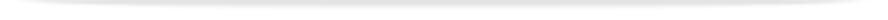units

MTE2547

Faculty of Engineeringprint version

This unit entry is for students who completed this unit in 2016 only. For students planning to study the unit, please refer to the unit indexes in the the current edition of the Handbook. If you have any queries contact the managing faculty for your course or area of study.

# MTE2547 - Structure-property relationships in materials

## 6 points, SCA Band 2, 0.125 EFTSL

Refer to the specific census and withdrawal dates for the semester(s) in which this unit is offered.

Faculty

Engineering

Organisational Unit

Department of Materials Science and Engineering

Coordinator(s)

Offered

Clayton

• Second semester 2016 (Day)

### Synopsis

The unit shows how the properties of materials and their structure can be mathematically analysed. Students will apply mathematical techniques to solve problems in various materials engineering fields. Examples of mechanical and electrical properties of materials are examined by the application of matrix operations. Heat transfer and diffusion in materials processing are used as exemplars of partial differential equations and boundary value problems. The error function is introduced. Finite difference methods are explored in relation to heat transfer and diffusion problems, basic curve fitting is introduced as a way of modelling a material's response to deformation. The statistical treatment of experimental data is presented. The distribution of errors for discrete and continuous data are analysed via the Binomial, Poisson and Normal distributions. Statistical testing and fitting of data and various forms of least square fitting of data is introduced. The applicability of non-parametric statistics is applied to a range of non-ordinal data. Problems are analysed using Excel and Matlab.

### Outcomes

To develop:

1. An understanding of the mathematical analysis of structure-property relationships in materials engineering

1. The ability to apply matrix operations to analyse material properties governed by material orientation

1. The ability to apply partial differential equations and their solution to practical heat transfer and diffusion problems in materials systems

1. A basic understanding of numerical methods

1. An understanding of the numerical treatment of experimental errors

1. Statistical data analysis and evaluation of the significance of the analysis

1. Skills in the use of Excel and Matlab software

### Assessment

Assignments: 40%
Laboratory class: 10%
Examination (3 hours): 50%